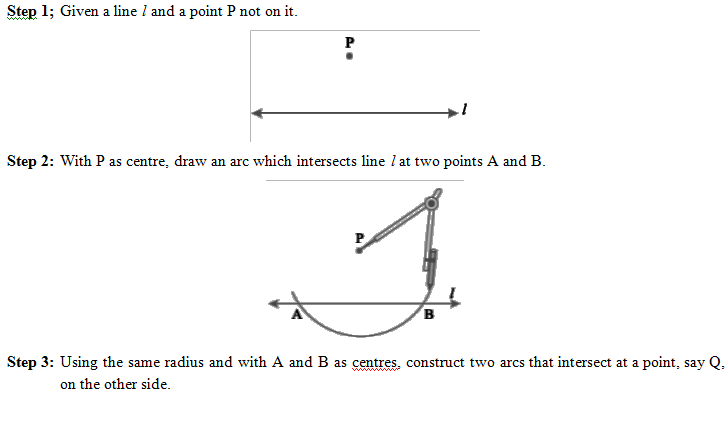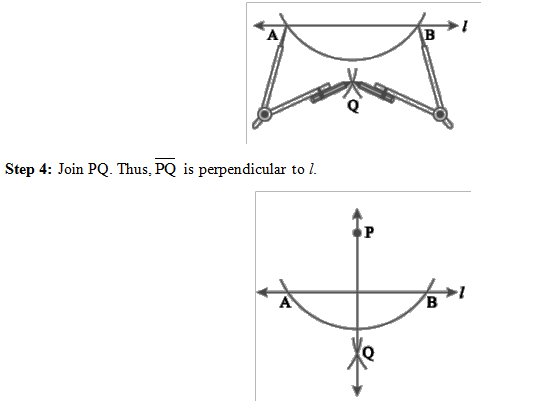# Construction of Line Segments

## CONSTRUCTION OF LINE SEGMENTS

Steps of construction:

• Step 1: Mark a point ‘A’ on the paper and place the ruler on the paper is such a way that zero mark of ruler coincides with ‘A’.
• Step 2: Mark another point ‘B’ against the mark on the ruler which indicates the given length.
• Step 3: Join A and B with pencil. The line segment AB so obtained is the required segment.

### Construction of the segment by using compasses:

In order to construct a line segment of given length using compasses, we follow the following steps.

Steps of construction:

• Step 1: Obtain the length of the line segment.
• Step 2: Mark a point A and draw a line passing through this point.
• Step 3:Place the metal point of the compasses on zero mark of ruler and open out it such that pencil and point on the mark that indicates the length of line segment.
• Step 4: Transfer the compasses as it is to the line l so that the metal points is on A.
• Step 5: With the pencil point make a small stroke on l so as to cut it at B.
• Step 6: The segment AB so obtained is the required segment of the given length.

Perpendicular to a line through a point on it

Method of ruler and set-square

Step of construction

Step 1: A line l and a point P are given. Note that P is on the line l.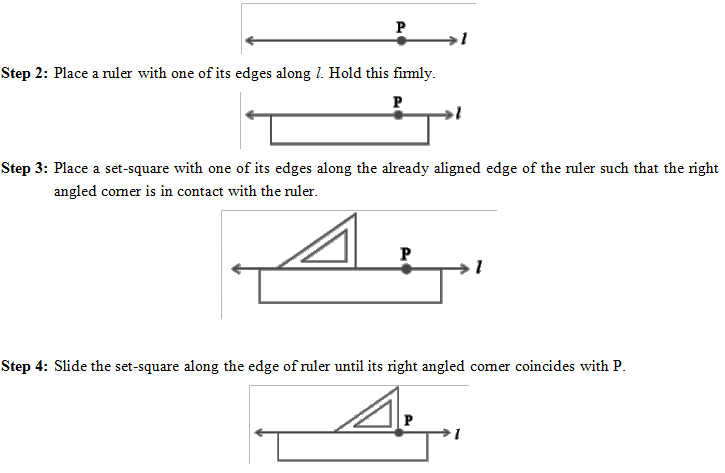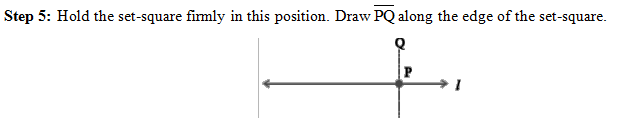Method of ruler and compasses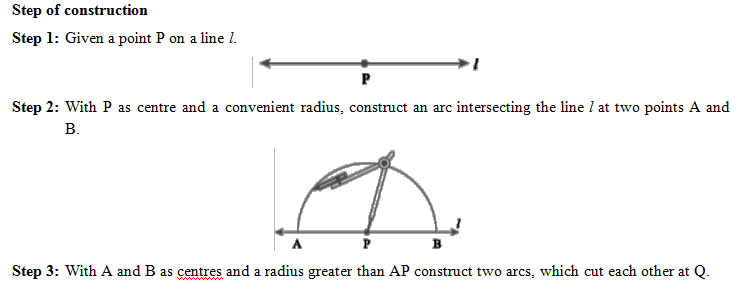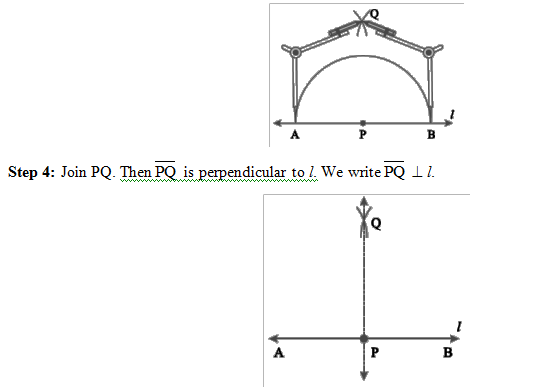### Perpendicular to a line through a point not on it

Method of ruler and set-square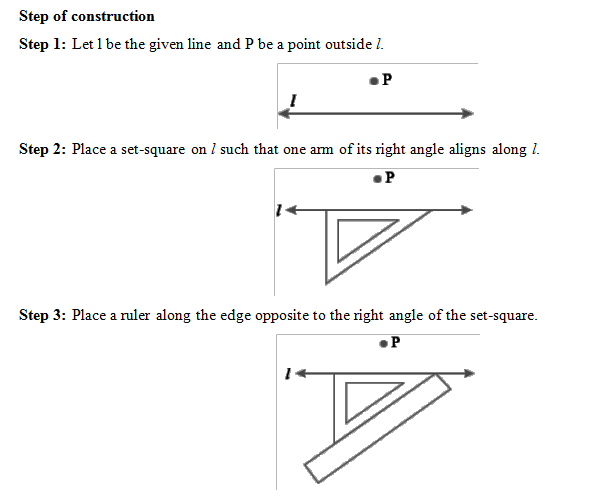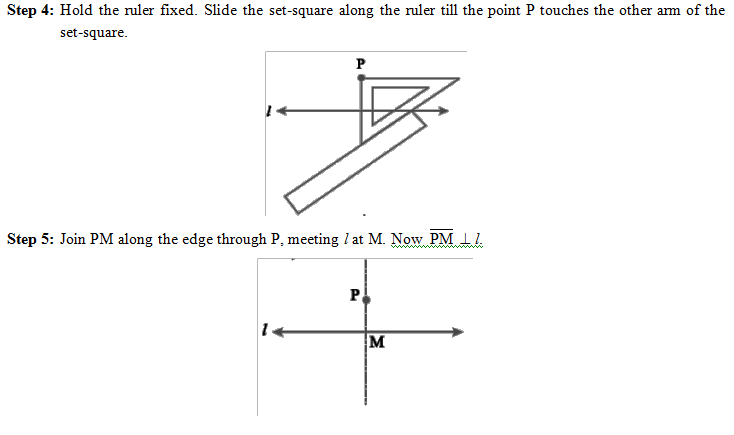Method of ruler and set-square

Step of construction